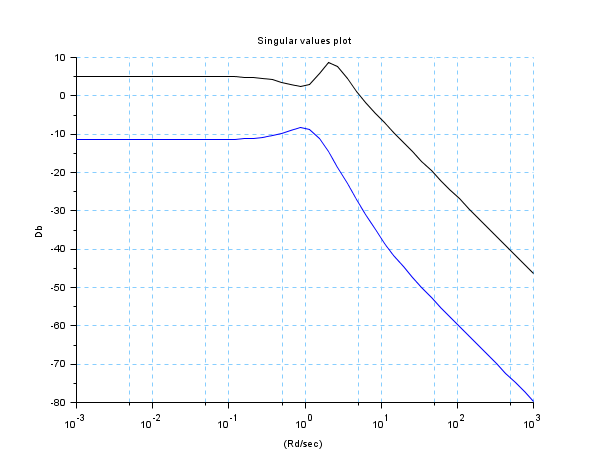Scilab Home page | Wiki | Bug tracker | Forge | Mailing list archives | ATOMS | File exchange
Change language to: Français - Português - 日本語 - Русский

See the recommended documentation of this function

# svplot

singular-value sigma-plot

### Syntax

`[SVM]=svplot(sl,[w])`

### Arguments

sl

`syslin` list (continuous, discrete or sampled system)

w

real vector (optional parameter)

### Description

computes for the system `sl=(A,B,C,D)` the singular values of its transfer function matrix:

```G(jw) = C(jw*I-A)B^-1+D
or
G(exp(jw)) = C(exp(jw)*I-A)B^-1+D
or
G(exp(jwT)) = C(exp(jw*T)*I-A)B^-1+D```

evaluated over the frequency range specified by `w`. (T is the sampling period, `T=sl('dt')` for sampled systems).

`sl` is a `syslin` list representing the system `[A,B,C,D]` in state-space form. `sl` can be continuous or discrete time or sampled system.

The `i`-th column of the output matrix `SVM` contains the singular values of `G` for the `i`-th frequency value `w(i)`.

`SVM = svplot(sl)`

is equivalent to

`SVM = svplot(sl,logspace(-3,3))  (continuous)`
`SVM = svplot(sl,logspace(-3,%pi)) (discrete)`

### Examples

```x = logspace(-3,3);
y = svplot(ssrand(2,2,4),x);
clf()
plot2d("ln", x, 20*log(y')/log(10))
xgrid(12)
xtitle("Singular values plot","(Rd/sec)", "Db");```